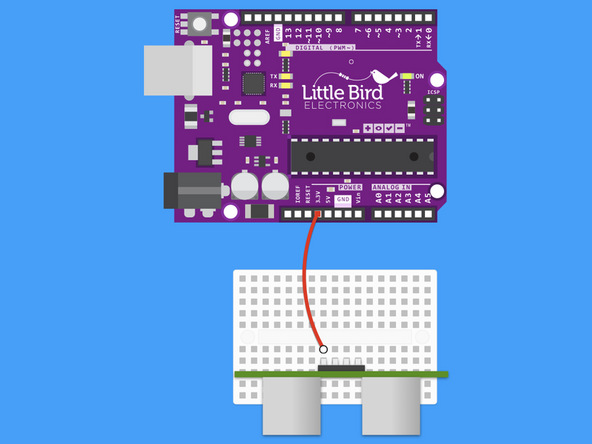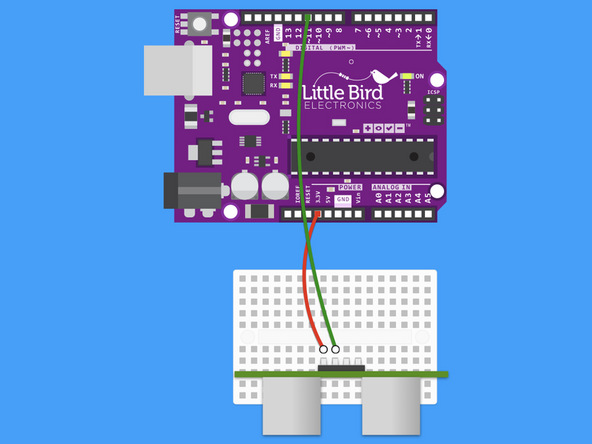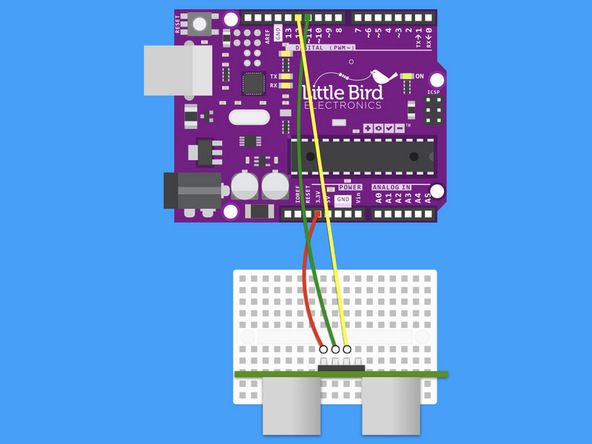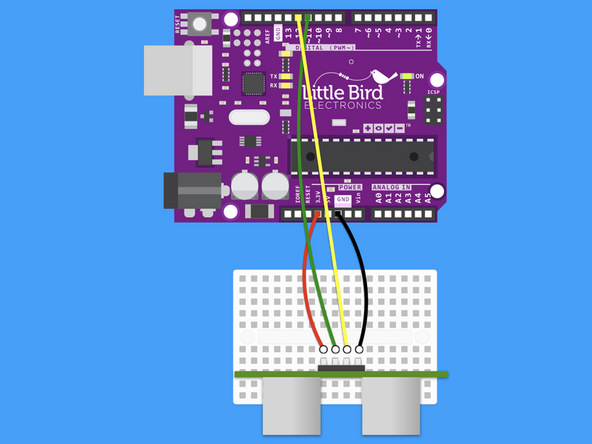## Introduction

An ultrasonic distance sensor measures distance by using ultrasonic waves, and so it can be used for proximity sensing.

In this guide, you will learn to use an ultrasonic distance sensor with a 100% Arduino-compatible board.

After completing this guide, you will gain a basic understanding on how to use an ultrasonic distance sensor and incorporate it into your next Arduino projects.

1. ## Step 1 Connect Arduino 5V to the Ultrasonic Range Finder• Connect Arduino 5V to the Ultrasonic Range Finder.

2. ## Step 2 Connect Arduino Digital 12 to Trig Pin• Connect Arduino Digital 12 to the Ultrasonic Range Finder's Trig Pin.

3. ## Step 3 Connect Arduino Digital 11 to Echo Pin• Connect Arduino Digital 11 to the Ultrasonic Range Finder's Echo Pin.

4. ## Step 4 Connect Arduino GND to the GND• Connect Arduino GND to the Ultrasonic Range Finder's GND.

5. ## Step 5 Measure the distance

``````// defines pins numbers
const int trigPin = 11;
const int echoPin = 12;

// defines variables
long duration;
int distance;

void setup() {
pinMode(trigPin, OUTPUT); // Make the trigPin an OUTPUT
pinMode(echoPin, INPUT); // Make the echoPin an INPUT
Serial.begin(9600); // Setup the Serial Port
}

void loop() {

// Clear the trigPin
digitalWrite(trigPin, LOW);
delayMicroseconds(2);

// Send a pulse by setting the trigPin Higj for 10 Micro seconds
digitalWrite(trigPin, HIGH);
delayMicroseconds(10);
digitalWrite(trigPin, LOW);

// Read the length of time for the sound wave to return
duration = pulseIn(echoPin, HIGH);

// Calculating the total distance from the object
distance = duration * 0.034 / 2;

// Prints the distance to the Serial Port
Serial.print("The Distance Is: ");
Serial.println(distance);
}``````
• This code will measure the distance from an object and print out the value to your Serial Monitor.

• Copy and paste the code into your Arduino Software.